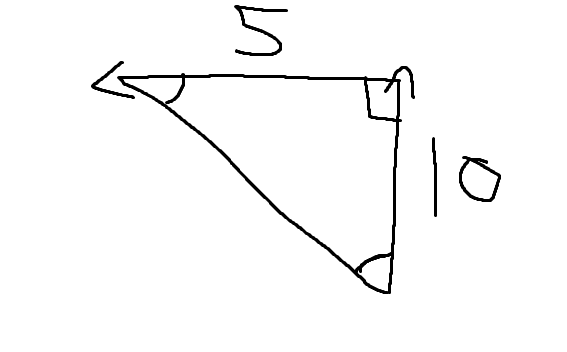# Very quick easy Trig/Angle question

• Pablo1122

## Homework Statement

The river flows 5km/hr in the west direction. The boat that has a speed of 10km/hr. It starts from the south bank to the north. Then the question is to essentially find the angle theta.

## Homework Equations

Tan-1(5/10) or Tan-1(10/5)

## The Attempt at a Solution

Tan^-1(5/10) or Tan^-1(5/10).

So basically the answer is asking to find the angle theta, when drawing the triangle I get this.Now basically I'm confused on what angle to take. If I take the top left angle then I would get tan^-1(10/5) but, if I take the bottom right get tan^-1(5/10).

All the answers provided are in terms of "east of north". If I look at this logically, north east is the top right but the east of north would be the angle at the bottom right. I'm just trying to know which one would be correct.

East of north is in the upper right quadrant. North of east is also in the upper right quadrant. Why can't you give the answer in terms of "north of west" or "west of north"?

East of north is in the upper right quadrant. North of east is also in the upper right quadrant. Why can't you give the answer in terms of "north of west" or "west of north"?
It was a multiple choice question and all the answers were written as "east of north" and one was "cannot be done without knowing width of the river".

Okay, I see. Was there a negative answer?

Okay, I see. Was there a negative answer?
No, there were no negative answers.

Well, was there an answer between 270 and 360?

Well, was there an answer between 270 and 360?
No there was not.

Please give the complete statement of the problem as it was given to you, as well as all of the choices you were given.

I suspect that they may be asking for the heading required for the boat to travel directly North.

•TomHart
If I take the top left angle then I would get tan^-1(10/5) but, if I take the bottom right get tan^-1(5/10).

That statement is correct. What makes the most sense to me is the bottom right angle that you drew, which you can find by tan-1(5/10). Whatever that turns out to be, I would describe that angle as "west of north".

That statement is correct. What makes the most sense to me is the bottom right angle that you drew, which you can find by tan-1(5/10). Whatever that turns out to be, I would describe that angle as "west of north".
ya that's what I did on the exam. I just put that answer to "east of north" which was on of the listed answers. It makes the most sense but it's not east of north so I was confused.

Pablo1222, please answer SammyS's request to give the complete problem statement (and choices). I thought he had a good point.

Please give the complete statement of the problem as it was given to you, as well as all of the choices you were given.

I suspect that they may be asking for the heading required for the boat to travel directly North.

This is based off memory of the exam. This is what I remember

The river flows 5km in the west direction. A boat which has a speed of 10km/hr traveling from the southbank to the north. Then I'm not sure how it was written but it essentially said to find the angle. The answers were all in degreee. Answers I remeber were, 27 degrees, "can't be solved without knowing width", 63 degrees. Those are all the ones I can remember, there were two more.

Pablo1222, please answer SammyS's request to give the complete problem statement (and choices). I thought he had a good point.

http://i.imgur.com/wv2YPjF.png

Not sure if this helps you at all.

Well, something is not right with the problem as it relates to the possible answers. Based on the problem you wrote, the answer looks like it should be 27° west of north, or 63° degrees north of west. And if you wanted to put the answer in terms of east of north, the answer should be -27° east of north.

...

Answers I remember were, 27 degrees, "can't be solved without knowing width", 63 degrees. Those are all the ones I can remember, there were two more.
You would probably remember 30°, if that was one of the choices.